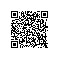# Tensorflow日常随笔(一)

TensorFlow is an end-to-end open source platform for machine learning

TensorFlow makes it easy for beginners and experts to create machine learning models. See the sections below to get started.

https://www.tensorflow.org/tutorials

Tutorials show you how to use TensorFlow with complete, end-to-end examples

https://www.tensorflow.org/guide

Guides explain the concepts and components of TensorFlow.

#### For beginners

The best place to start is with the user-friendly Sequential API. You can create models by plugging together building blocks. Run the “Hello World” example below, then visit the tutorials to learn more.

To learn ML, check out our education page. Begin with curated curriculums to improve your skills in foundational ML areas.

import tensorflow as tf
mnist = tf.keras.datasets.mnist

x_train, x_test = x_train / 255.0, x_test / 255.0

model = tf.keras.models.Sequential([
tf.keras.layers.Flatten(input_shape=(28, 28)),
tf.keras.layers.Dense(128, activation='relu'),
tf.keras.layers.Dropout(0.2),
tf.keras.layers.Dense(10, activation='softmax')
])

loss='sparse_categorical_crossentropy',
metrics=['accuracy'])

model.fit(x_train, y_train, epochs=5)
model.evaluate(x_test, y_test)

#### For experts

class MyModel(tf.keras.Model):
def __init__(self):
super(MyModel, self).__init__()
self.conv1 = Conv2D(32, 3, activation='relu')
self.flatten = Flatten()
self.d1 = Dense(128, activation='relu')
self.d2 = Dense(10, activation='softmax')

def call(self, x):
x = self.conv1(x)
x = self.flatten(x)
x = self.d1(x)
return self.d2(x)
model = MyModel()

logits = model(images)
loss_value = loss(logits, labels)
optimizer.apply_gradients(zip(grads, model.trainable_variables))

### Learn about the relationship between TensorFlow and Keras

TensorFlow's high-level APIs are based on the Keras API standard for defining and training neural networks. Keras enables fast prototyping, state-of-the-art research, and production—all with user-friendly APIs.

## Solutions to common problems

https://www.tensorflow.org/tutorials/keras/classification

https://www.tensorflow.org/tutorials/generative/dcgan

https://www.tensorflow.org/tutorials/text/nmt_with_attention

## News & announcements

Check out our blog for additional updates, and subscribe to our monthly TensorFlow newsletter to get the latest announcements sent directly to your inbox.使用钉钉扫一扫加入圈子
+ 订阅